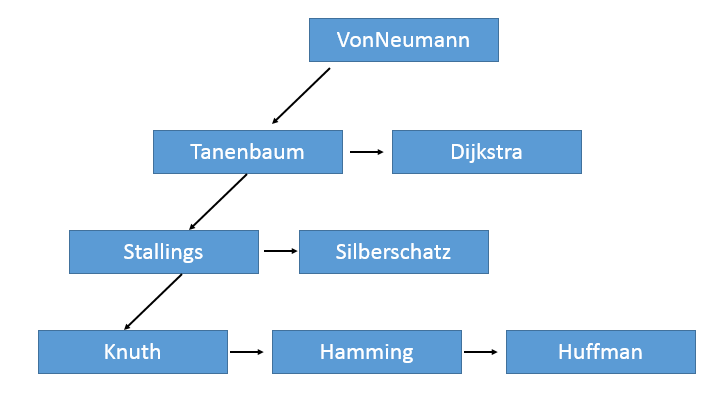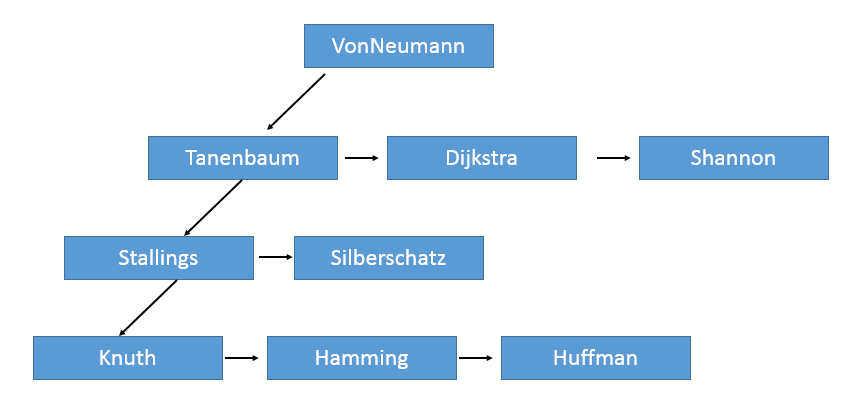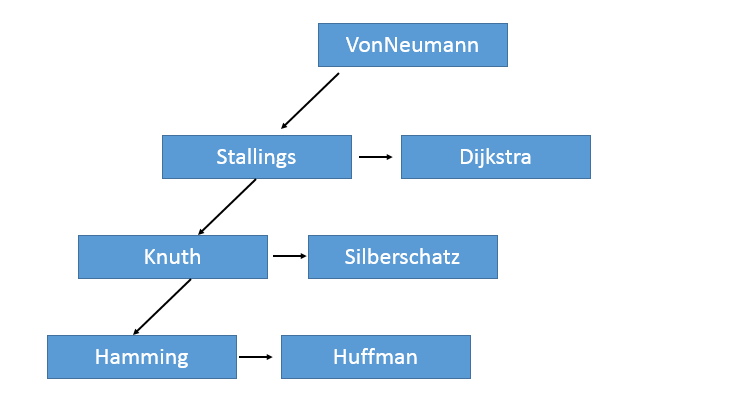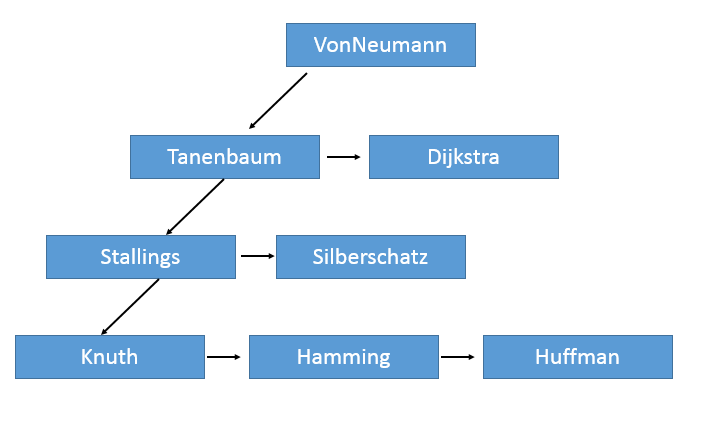<meter id="xzewj"></meter>
1. <noscript id="xzewj"></noscript>
2. <video id="xzewj"></video>
<table id="xzewj"></table>
<noscript id="xzewj"></noscript>
1. <pre id="xzewj"></pre>0315-5269188

工作時間

09:00 - 17:00

數字生態·驅動未來 企業數字化管理解決方案
當前位置：首頁 > 技術文檔

## 描述

### 對于一間公司來說，它成立之時所做的第一件事恐怕就是任命CEO了。之后，CEO就會開始雇用員工，也會有員工離職去別的公司。假設公司中的每一個員工（包括 CEO在內）都可以直接雇用新的員工，而公司中的所有員工（包括CEO）也都可能會跳槽離開，則某公司在成立一段時間之后的的組織結構如圖1VonNeumann是公司的CEO，他直接雇用了兩個人，分別是Tanenbaum和Dijkstra。在公司中，某員工的幾個直接下屬，他們的職位是由員工們的工齡決定的，在圖中即表現為從從左至右職位越來越低，譬如Tanenbaum的職位就比Dijkstra要高。

當一個員工雇用了新的下屬時，該下屬在該員工雇傭的所有下屬中，職位是最低的。假設VonNeumann又雇用了Shannon，則VonNeumann的三名下屬職位從高到低分別是Tanenbaum、Dijkstra和Shannon。

當公司中的員工離職，則有兩種情況。若他沒有雇用任何下屬，則他會被從公司的組織結構中拿掉。若他有直接的下屬，則他的直接下屬中職位較高的人，會升職并補上缺位。而該下屬若也有下屬，則他的下屬中職位最高的，會補上他升值后空出的位子。以此類推，直到某個尚未雇用下屬的員工升職。

假設圖1中的Tanenbaum跳槽離開了，則Stallings會補上它的位置，而Knuth會補上Stallings的位置。圖2圖3分別展示了基于圖1的兩種的操作的結果。圖2： VonNeumann雇用了Shannon。圖3： Tanenbaum跳槽。### 輸入

輸入的第一行是 CEO 的姓名。題目中所有的人名的長度都在2-20之間，且由大小寫字母、數字和短線（減號）組成。每個名字都包含至少一個大寫和一個小寫字母。

在第一行后會有很多行內容，他們由如下的規則構成：

• [老員工] hires [新員工]
• fire [老員工]
• print

[老員工] 是已經在公司工作的人的名字，而[新員工]是即將被雇用的員工的名字。以上三種規則組成的內容可能會按照任何順序出現。但公司中會至少有一名員工（CEO），且公司的規模最大不會超過 1000 人。

### 輸出

對于每一個打印命令，按照如下規則輸出當前公司的結構信息：

• 每一行包含一個人名
• 第一行是CEO的名字，從第一列開始
• 對于圖4形式的樹• 輸出如下
• ```VonNeumann
+Tanenbaum
++Stallings
+++Knuth
+++Hamming
+++Huffman
++Silberschatz
+Dijkstra```
• *關于print的說明：從ceo開始打印，職位每降低一個等級（參考圖片和輸出），在名字前面加上一個加號。先序（自己->左枝->右枝）輸出整棵樹的節點。整棵樹遍歷完之后輸出一行"---------"(具體長度看測試用例1)。
• 輸入以EOF結束

學長代碼鏈接：1. 點擊打開鏈接

2. 點擊打開鏈接

這個題我花了很長時間，其實錯誤對我而言確實是不明顯：

我的正確代碼如下：

1.
`#include<stdio.h>`
2.
`#include<stdlib.h>`
3.
`#include<string.h>`
4.

5.
`typedef struct node{`
6.
`char name;`
7.
`struct node * left;`
8.
`struct node * right;`
9.
`}NODE;`
10.

11.
`NODE **Father=NULL;`
12.
`NODE **Delete=NULL;`
13.
`NODE *CEO=NULL;`
14.
`void print(NODE *ptr,int dep)`
15.
`{`
16.
`if(ptr!=NULL)`
17.
`{`
18.
`for(int i=0;i<dep;i++)`
19.
`printf("+");`
20.
`printf("%s\n",ptr->name);`
21.
`}`
22.
`if(ptr->left!=NULL)`
23.
`print(ptr->left,dep+1);`
24.
`if(ptr->right!=NULL)`
25.
`print(ptr->right,dep); `
26.
`}`
27.

28.
`void Build(NODE **ptr,char *s)`
29.
`{`
30.
`while((*ptr)!=NULL)`
31.
`ptr=&((*ptr)->right);`
32.
`(*ptr)=(NODE*)malloc(sizeof(NODE));`
33.
`strcpy((*ptr)->name,s);`
34.
`(*ptr)->right=NULL; `
35.
`(*ptr)->left=NULL;`
36.
`}`
37.
`int Hire(NODE **ptr,char *p,char *q)`
38.
`{`
39.
`if(*ptr==NULL) return 0;`
40.
`if(strcmp(p,(*ptr)->name)==0)`
41.
`{`
42.
`if((*ptr)->left==NULL)`
43.
`{`
44.
`(*ptr)->left=(NODE*)malloc(sizeof(NODE));`
45.
`(*ptr)->left->left=NULL;`
46.
`(*ptr)->left->right=NULL;`
47.
`strcpy((*ptr)->left->name,q);`
48.
`}`
49.
`else`
50.
`{`
51.
`Build(&((*ptr)->left),q);`
52.
`}`
53.
`return 1;`
54.
`}`
55.
`else`
56.
`{`
57.
`if((*ptr)->left!=NULL)`
58.
`Hire(&((*ptr)->left),p,q);`
59.

60.
`if((*ptr)->right!=NULL)`
61.
`Hire(&((*ptr)->right),p,q);`
62.
`}`
63.
`return 0;`
64.
`}`
65.

66.
`void Search(NODE **ptr, char *s)`
67.
`{`
68.
`if((*ptr)==NULL) return;`
69.
`if((*ptr)->left!=NULL&&strcmp((*ptr)->left->name,s)==0)`
70.
`{`
71.
`Father=ptr;`
72.
`Delete=&((*ptr)->left);`
73.
`return;`
74.
`}`
75.
`if((*ptr)->right!=NULL&&strcmp((*ptr)->right->name,s)==0)`
76.
`{`
77.
`Father=ptr;`
78.
`Delete=&((*ptr)->right);`
79.
`return;`
80.
`}`
81.
`if((*ptr)->left!=NULL)`
82.
`Search(&((*ptr)->left),s);`
83.
`if((*ptr)->right!=NULL) `
84.
`Search(&((*ptr)->right),s);`
85.
`return;`
86.
`}`
87.

88.
`void Fire(char *s)`
89.
`{`
90.

91.

92.
`if(strcmp(s,CEO->name)==0 && CEO->left!=NULL)`
93.
`{`
94.
`char str;`
95.
`strcpy(str,CEO->name);`
96.
`strcpy(CEO->name,CEO->left->name);`
97.
`strcpy(CEO->left->name,str);`
98.
`}`
99.
`else if(strcmp(s,CEO->name)==0 && CEO->right!=NULL)`
100.
`{`
101.
`char str;`
102.
`strcpy(str,CEO->name);`
103.
`strcpy(CEO->name,CEO->right->name);`
104.
`strcpy(CEO->right->name,str);`
105.
`}`
106.
`else;`
107.
`Father=NULL; Delete=NULL;`
108.
`Search(&CEO,s);`
109.
`if(Father==NULL)`
110.
`{`
111.
`return; `
112.
`}`
113.
`else`
114.
`{`
115.
`if((*Father)->left== *Delete)`
116.
`{`
117.
`if((*Delete)->left==NULL)`
118.
`{`
119.
`(*Father)->left=(*Delete)->right;`
120.
`return;`
121.
`}`
122.
`else`
123.
`{`
124.
`char str;`
125.
`strcpy(str,(*Delete)->name);`
126.
`strcpy((*Delete)->name,(*Delete)->left->name);`
127.
`strcpy((*Delete)->left->name,str);`
128.
`Fire(s);`
129.
`}`
130.
`}`
131.
`else if((*Father)->right== *Delete)`
132.
`{`
133.
`if((*Delete)->left==NULL)`
134.
`{`
135.
`(*Father)->right=(*Delete)->right;`
136.
`return;`
137.
`}`
138.

139.
`else if((*Delete)->right==NULL)`
140.
`{`
141.
`(*Father)->right=(*Delete)->left;`
142.
`return;`
143.
`}`
144.
`else`
145.
`{`
146.
`char str;`
147.
`strcpy(str,(*Delete)->name);`
148.
`strcpy((*Delete)->name,(*Delete)->left->name);`
149.
`strcpy((*Delete)->left->name,str);`
150.
`Fire(s);`
151.
`}`
152.
`}`
153.
`else;`
154.
`}`
155.

156.
`}`
157.
`int main()`
158.
`{`
159.
`CEO=(NODE*)malloc(sizeof(NODE));`
160.
`CEO->left=NULL;`
161.
`CEO->right=NULL;`
162.
`int input;`
163.
`char inputs,prase1,prase2,prase3;`
164.
`scanf("%s",CEO->name);`
165.
`while(gets(inputs)!=NULL)`
166.
`{`
167.
`input=sscanf(inputs,"%[^ ] %[^ ] %[^ ]",prase1,prase2,prase3);`
168.
`if(input==1)`
169.
`{`
170.
`print(CEO,0);`
171.
`printf("------------------------------------------------------------\n");`
172.
`}`
173.
`else if(input==2)`
174.
`Fire(prase2);`
175.
`else if(input==3)`
176.
`Hire(&CEO,prase1,prase3);`
177.
`}`
178.
`return 0; `
179.
`} `

錯誤的地方是Fire函數，錯誤代碼如下：

1.
`void Fire(char *s)`
2.
`{`
3.
`if(strcmp(s,CEO->name)==0 && CEO->left!=NULL)`
4.
`{`
5.
`char str;`
6.
`strcpy(str,CEO->name);`
7.
`strcpy(CEO->name,CEO->left->name);`
8.
`strcpy(CEO->left->name,str);`
9.
`}`
10.
`else if(strcmp(s,CEO->name)==0 && CEO->right!=NULL)`
11.
`{`
12.
`char str;`
13.
`strcpy(str,CEO->name);`
14.
`strcpy(CEO->name,CEO->right->name);`
15.
`strcpy(CEO->right->name,str);`
16.
`}`
17.
`else;`
18.
`Father=NULL; Delete=NULL;`
19.
`Search(&CEO,s);`
20.
`if(Father==NULL)`
21.
`{`
22.
`return; `
23.
`}`
24.
`else`
25.
`{`
26.
`if((*Father)->left== *Delete) // *1*`
27.
`{`
28.
`if((*Delete)->left==NULL)`
29.
`{`
30.
`(*Father)->left=(*Delete)->right;`
31.
`return;`
32.
`}`
33.
`else`
34.
`{`
35.
`char str;`
36.
`strcpy(str,(*Delete)->name);`
37.
`strcpy((*Delete)->name,(*Delete)->left->name);`
38.
`strcpy((*Delete)->left->name,str);`
39.
`Fire(s);`
40.
`}`
41.
`}`
42.
`if((*Father)->right== *Delete) //錯誤的地方`
43.
`{`
44.
`if((*Delete)->left==NULL)`
45.
`{`
46.
`(*Father)->right=(*Delete)->right;`
47.
`return;`
48.
`}`
49.

50.
`else if((*Delete)->right==NULL)`
51.
`{`
52.
`(*Father)->right=(*Delete)->left;`
53.
`return;`
54.
`}`
55.
`else`
56.
`{`
57.
`char str;`
58.
`strcpy(str,(*Delete)->name);`
59.
`strcpy((*Delete)->name,(*Delete)->left->name);`
60.
`strcpy((*Delete)->left->name,str);`
61.
`Fire(s);`
62.
`}`
63.
`}`
64.
`} `
65.
`}`

錯誤之處在于程序進入*1*處的  if語句塊中，將節點已經刪除，但是程序依舊進行 *2* 處條件判斷，但此時Delete指針所指的區域已經被刪除，所以程序會提示內存訪問沖突。

這件事提示我如果已經刪除了一個節點，就不能再對它進行判斷或者操作，否則會有內存訪問沖突的錯誤。地址：唐山市新華西道大洋商廈4層

電話：0315-5269188

電話：18633118288

郵箱：52731887@qq.com

為您提供專業的 唐山網頁設計 唐山網站建設 唐山網站制作 等優質的唐山網絡布線服務

歡迎國內外/唐山網站建設,唐山網絡布線,唐山網站制作,網站設計服務公司同行與我們建立友情鏈接

版權所有

2. 唐山赫鳴科技有限公司 冀ICP備11004205號-1
3. 99国精品午夜福利视频不卡99,特级欧美bbbbxxxx,吃奶摸下激烈床震视频大尺度,国产aⅴ精品一区二区三区尤物